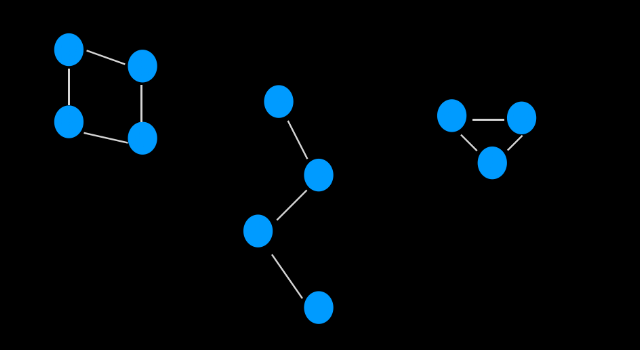Things we will discuss here are,

• Connected Component
• Counting the number of Connected components in a Graph.
• Articulation Points
• Bridges
• Importance of Bridges and Articulation Points.

Let us start by defining the Connected Component,

## Connected Component

A connected component of an undirected graph is a subgraph in which any two vertices are connected to each other by a path and which is connected to no additional vertices in the subgraphs. For example in the given image has three connected components.Fig 1: Graph with 3 componentCounting the number of Connected Components.

Now we will be discussing an Algorithm to find the number of Connected components. As we know that to get the total number of Connected Component we need to traverse the whole graph and for this traversal, we have already been discussed two algorithms called Depth-first Search and Breadth-first Search.

Here we will be manipulating Depth-first Search Algorithm to get the number of connected components.Looking into the Depth-first Search algorithm we know that if the graph is connected then we can traverse the whole graph from a single source node but in this case, we would not be able to traverse the whole graph using one DFS.

At a time DFS will traverse just one component as there is no path from that one component to the next component. From this, we get that the number of Connected Component is equal to the number of DFS run we make to traverse the whole Graph.

Implementation in C++

``````#include<bits/stdc++.h>
#define ll long long int
#define mod 1000000007
using namespace std;

void dfs(bool visited[], vector<int> adj[], int s){
visited[s]=true;
if(visited[u]) continue;
visited[u]=true;
}
}

void solve(){
int n,m; cin>>n>>m; // n-number of nodes(1-n), m-number of edges for an undirected graph
for(int i=0; i<m; i++){
int u,v; cin>>u>>v;
}

bool visited[n+1];
memset(visited,false,sizeof visited);

int number_of_connected_component=0; // will be equal to number of times
//we call dfs() to completely traverse the graph.
for(int i=1; i<=n; i++){
if(!visited[i]){
number_of_connected_component++;
}
}

cout<<"The number of connected compoenet in the given graph: "<<number_of_connected_component<<"\n";
}

int main(){
ios_base::sync_with_stdio(false);
cin.tie(NULL);
solve();
return 0;
}``````

Input:

``````10 11
1 2
2 3
1 3
1 4
2 4
5 6
6 7
5 7
8 9
9 10
8 10``````

Output:

``The number of connected compoenet in the given graph: 3``

## Articulation Points

Articulation points are those vertexes in a graph after whose removal with all its associated edges (Edges that are connected with this node) the number of the component gets increased. As we can see from this graph,

Here if we remove node 2, the number of connected components will be 2, the first will be containing node 1 and the second component will have node 3,4. Similarly, if we remove node 3 then also we will get two connected components. The basic approach to find a number of Articulation Points are

• First of all, find the number of connected components in the original graph (originalCount).
• Then remove i-th vertex and its associated edges and find number of connected component (modifiedCount) one at a time, i=1,2,3, … n.
• if(modifiedCount>originalCount) then i-th vertex is Articulation Points else not.
• The running time of this algorithm is O ( V * (V+E) ) as for DFS running time is O(V+E) and we will have to run it for V times.
• In the coming article, we will also explore the back edge concept to find all articulation points in O(V+E) time complexity.

## Bridges

An edge in a graph between vertices u and v are called a Bridge if after removing it, there will be no path left between u and v. Its definition is very similar to that of Articulation Points. The basic approach to find all the bridges in a given graph is to check for every edge if it is a bridge or not, by first removing it and then checking if the vertices that it was connecting are still connected or not.

• Example: In Fig 2 edges (1,2), (2,3) and (3,4) are bridges.
• The running time of this algorithm is O (E * (V+E) ).
• In the coming article, we will also explore the back edge concept to find all bridges in O(V+E) time complexity.

## Importance of Bridges and Articulation Points.

Bridges and Articulation Points represent vulnerabilities in the given network. For example, if we consider Fig 2. then all the three edges are bridges as after removal of anyone edges disconnects the network. And in the same way node, 2 and 3 are articulation points as removal of these also disconnects the network.

## Practice Problem

That’s all for this article, in the next article we will be discussing Application of DFS – Cycle Detection and problems related to them. Before moving to the next session do practice the above problems ( If you stuck then do comment Question Number for Solution and your approach so that other programmer or we can help you ).

If you have some content OR material or want to add something related to this then do share them through the comment section for which we will be thankful to you.

Thank You
With 💙 by Learn DSA